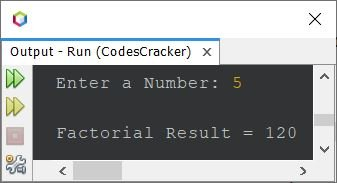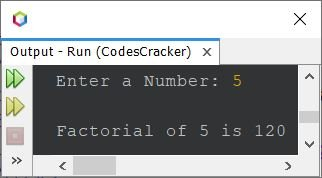# Java Program to Find the Factorial of a Number

This article covers a program in Java that finds and prints the factorial of a number. The factorial of a number n is calculated as n * (n-1) * (n-2) * ... * 1. For example, the factorial of 5 is 5*4*3*2*1 or 120.

## Find the factorial of a number in Java using the for loop

The question is: write a Java program to find the factorial of a number. The number must be received by the user at the runtime of the program. The answer to this question is the program given below:

```import java.util.Scanner;

public class CodesCracker
{
public static void main(String[] args)
{
int num, i, fact=1;
Scanner s = new Scanner(System.in);

System.out.print("Enter a Number: ");
num = s.nextInt();

for(i=num; i>=1; i--)
{
fact = fact*i;
}

System.out.println("\nFactorial Result = " +fact);
}
}```

The sample run of the above Java program with user input 5 is shown in the snapshot given below:The above program can also be created in this way:

```import java.util.Scanner;

public class CodesCracker
{
public static void main(String[] args)
{
int fact=1;
Scanner s = new Scanner(System.in);

System.out.print("Enter a Number: ");
int num = s.nextInt();

for(int i=num; i>=1; i--)
fact = fact*i;

System.out.println("\nFactorial of " +num+ " is " +fact);
}
}```

Here is its sample run with the same user input as the previous program's sample run:## Find the factorial of a number in Java using the while loop

This program does the same job as the previous one, but was created using the while loop instead of the for

```import java.util.Scanner;

public class CodesCracker
{
public static void main(String[] args)
{
int fact=1;
Scanner s = new Scanner(System.in);

System.out.print("Enter a Number: ");
int num = s.nextInt();

int i = num;
while(i>=1)
{
fact = fact*i;
i--;
}

System.out.println("\nFactorial of " +num+ " is " +fact);
}
}```

The above program produces the same output as the previous program. In the above program, the following two statements:

```fact = fact*i;
i--;```

can also be replaced with a single statement given below:

`fact = fact*i--;`

In the above statement, the i-- means the current value of i gets used and then decremented because of a post-increment (--) operator used.

## Find the factorial in Java using a function

This program is created using a user-defined function named factorial() that takes an argument and returns the factorial result of its argument.

```import java.util.Scanner;

public class CodesCracker
{
public static void main(String[] args)
{
Scanner s = new Scanner(System.in);

System.out.print("Enter a Number: ");
int num = s.nextInt();

System.out.println("\nFactorial of " +num+ " is " +factorial(num));
}

public static int factorial(int n)
{
int fact = 1;
for(int i=n; i>=1; i--)
fact = fact*i;
return fact;
}
}```

## Find the factorial of a number in Java using recursion

Here is another Java program I've created to find the factorial of a number using recursion, or a recursive function. A recursive function is a function where the function calls itself from inside its definition.

```import java.util.Scanner;

public class CodesCracker
{
public static void main(String[] args)
{
Scanner s = new Scanner(System.in);

System.out.print("Enter a Number: ");
int num = s.nextInt();

System.out.println("\nFactorial of " +num+ " is " +factorial(num));
}

public static int factorial(int n)
{
if(n>=1)
return (n * factorial(n-1));
else
return 1;
}
}```

Again, the output produced by this Java program is the same as that of the previous program.

#### Same Program in Other Languages

Java Online Test

« Previous Program Next Program »